Want to share your content on R-bloggers? click here if you have a blog, or here if you don't.

I have written about the basics of Functional Data Analysis in three prior posts. In Post 1, I used the `fda` package to introduce the fundamental concept of using basis vectors to represent longitudinal or time series data as a curve in an abstract vector space. In Post 2, I continued to rely on the `fda` package to show basic FDA descriptive statistics. In Post 3, I introduced Functional PCA with the help of the `fdapace` package. In this post, I pick up where that last post left off and look at how one might explore a sparse, longitudinal data set with the FPCA tools provided in the `fdapace` package. I will begin by highlighting some of the really nice tools available in the `brolgar` package for doing exploratory longitudinal data analysis. While the first three posts made do with artificial data constructed with an algorithm for generating data from a Wiener Process, in this post I’ll use the `wages` data set available in `brolgar`.

`brolgar` is a beautiful, tidyverse inspired package, based on the `tsibble` data structure that offers a number of super helpful functions for visualizing and manipulating longitudinal data. See the arXiv paper Tierney, Cook and Prvan (2021) for an overview, and the seven package vignettes for examples. Collectively, these vignettes offer a pretty thorough exploration of the `wages` data set. Using `wages` for this post should provide a feeling for what additional insight FDA may have to offer.

### Longitudinal Data

Let’s look at the data. `wages` contains measurements on hourly wages associated with years of experience in the workforce along with several covariates for male high school dropouts who were between 14 and 17 years old when first measured. In what follows, I will use only`ln_wages`, the natural log of wages in 1990 dollars, and `xp` the number of years of work experience.

```library(brolgar)
library(fdapace)
library(tidyverse)
library(plotly)
dim(wages)
##  6402    9
## # A tsibble: 6 x 9 [!]
## # Key:       id 
##      id ln_wages    xp   ged xp_since_ged black hispanic high_grade
##   <int>    <dbl> <dbl> <int>        <dbl> <int>    <int>      <int>
## 1    31     1.49 0.015     1        0.015     0        1          8
## 2    31     1.43 0.715     1        0.715     0        1          8
## 3    31     1.47 1.73      1        1.73      0        1          8
## 4    31     1.75 2.77      1        2.77      0        1          8
## 5    31     1.93 3.93      1        3.93      0        1          8
## 6    31     1.71 4.95      1        4.95      0        1          8
## # … with 1 more variable: unemploy_rate <dbl>```

A summary of the variables shows that wages vary from 2 dollars per hour to a high of about 73 dollars per hour. Time varies between 0 and almost 13 years.

```wages %>% select(ln_wages, xp) %>% summary()
##     ln_wages           xp               id
##  Min.   :0.708   Min.   : 0.001   Min.   :   31
##  1st Qu.:1.591   1st Qu.: 1.609   1st Qu.: 3194
##  Median :1.842   Median : 3.451   Median : 6582
##  Mean   :1.897   Mean   : 3.957   Mean   : 6301
##  3rd Qu.:2.140   3rd Qu.: 5.949   3rd Qu.: 9300
##  Max.   :4.304   Max.   :12.700   Max.   :12543```

Next, we construct the `tsibble` data structure to make use of some of the very convenient `brolgar` sampling functions, and count the number of observations for each subject.

```wages_t <- as_tsibble(wages,
key = id,
index = xp,
regular = FALSE)

num_obs <- wages_t %>% features(ln_wages,n_obs)
summary(num_obs\$n_obs)
##    Min. 1st Qu.  Median    Mean 3rd Qu.    Max.
##    1.00    5.00    8.00    7.21    9.00   13.00```

Just like the `brolgar` package vignettes, we filter out subjects with less than 3 observations. Then we use the `sample_n_keys()` function to generate a random sample of 10 wages versus year’s experience curves and plot them.

```df <- wages_t %>%  add_n_obs() %>% filter(n_obs > 3)
set.seed(123)
df %>%
sample_n_keys(size = 10) %>%
ggplot(aes(x = xp,  y = ln_wages, group = id, color = id)) +
geom_line()````brolgar` makes it easy to generates lots of panels with small numbers of curves in order to get a feel for the data.

```df %>% ggplot(aes(x = xp, y = ln_wages, group = id, colour = id)) +
geom_line() +
facet_sample(n_per_facet = 3, n_facets = 20)```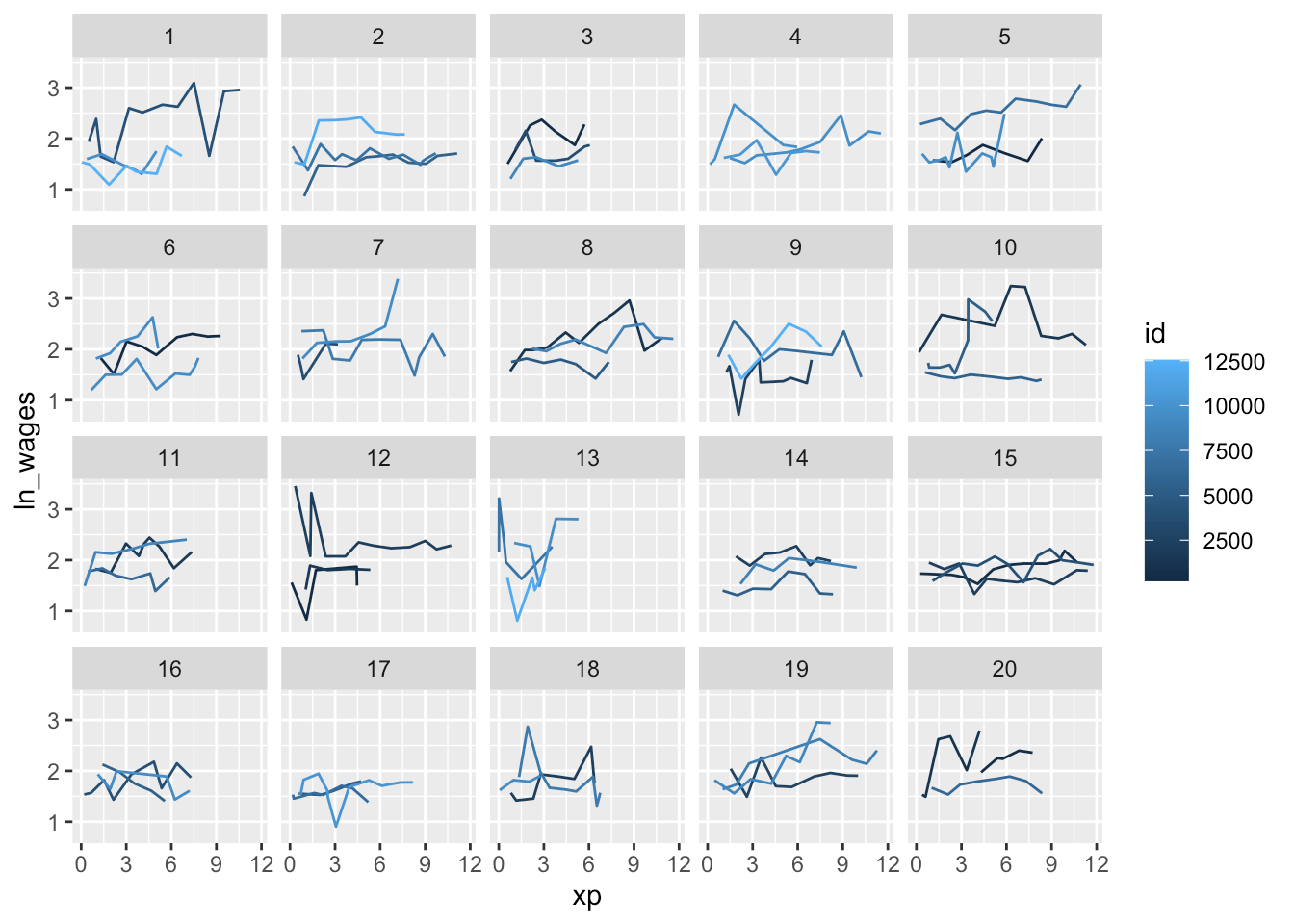Finally, for comparison with the plots produced by `fdapace` we plot the curves for the first two subjects in the `tsibble`.

```df  %>% filter(id == 31 | id == 36)  %>%
ggplot(aes(x = xp, y = ln_wages, group = id, color = id)) +
geom_line()```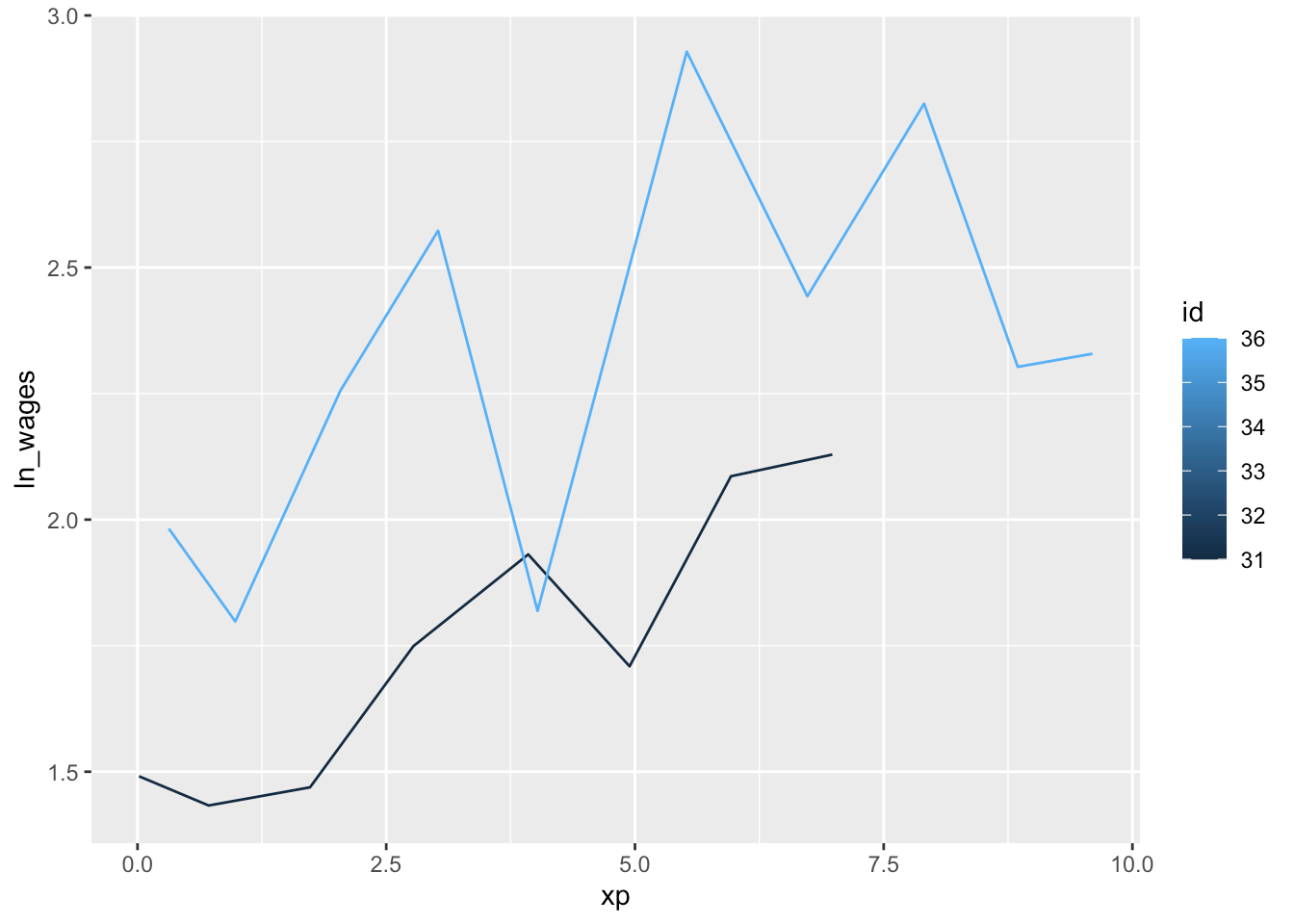We finish our initial look at the data by noting that we are really dealing with sparse data here. Some curves have only 4 measurements, no curve has more than 13 measurements, and all subjects were measured at different times. This is a classic longitudinal data set.

### Functional PCA

As I mentioned in my previous post, Principal Components by Conditional Expectation (PACE), described in Yao, Müller & Wang (2005), was designed for sparse data. The method works by pooling the data. Curves are not individually smoothed. Instead, estimates of the FPC scores are obtained from the entire ensemble of data. (See equation (5) in the reference above.)

The first step towards using FPCA functions in the `fdapace` package is to reshape the data so that the time and wages data for each subject are stored as lists in separate columns of a `tibble` where each row contains all of the data for a single id. (Standard `dplyr` operations might not work as expected on a `tsibble`.) The following code pulls just the required data into a `tibble` before the `dplyr` code in the somewhat untidy ‘for loop’ builds the data structure we will use for the analysis.

```df2 <- df %>% select(id, xp, ln_wages) %>% as_tibble()

uid <- unique(df2\$id)
N <- length(uid)
Wages <- rep(0,N)
Exp <- rep(0,N)

for (k in 1:N){
Wages[k] <-  df2 %>% filter(id == uid[k]) %>% select(ln_wages) %>% pull()  %>% list()
Exp[k]  <-   df2 %>% filter(id == uid[k]) %>% select(xp)  %>% pull() %>% list()
}
df3 <- tibble( uid, Wages, Exp )
glimpse(df3)
## Rows: 764
## Columns: 3
## \$ uid   <int> 31, 36, 53, 122, 134, 145, 155, 173, 207, 222, 223, 226, 234, 24…
## \$ Wages <list> <1.491, 1.433, 1.469, 1.749, 1.931, 1.709, 2.086, 2.129>, <1.98…
## \$ Exp   <list> <0.015, 0.715, 1.734, 2.773, 3.927, 4.946, 5.965, 6.984>, <0.31…```

he `FPCA()` function computes the functional principal components. Note that in the function call the input data are the two columns of lists we created above. The `dataType` parameter specifies the data as being sparse. `error = FALSE` means we are using a simple model that does not account for unobserved error. `kernel =`epan` means that the we are using the Epanechnikov for smoothing the pooled data to compute the mean and covariance. (For this data set, Epanechnikov seems to yield better results than the default Gaussian kernel.)

`res_wages <- FPCA(df3\$Wages,df3\$Exp, list(dataType='Sparse', error=FALSE, kernel='epan', verbose=TRUE))`

The plot method for the resulting `FPCA` object provides a visual summary of the results. Going clockwise from the upper left, the Design Plot shows that collectively the data are fairly dense over the support except at the upper range near twelve years. The computed mean function for the data shows a little dip in wages near the beginning and then a clear upward trend until it abruptly drops towards the end. The first three eigenfunctions are plotted at the bottom right, and the scree plot at the bottom left shows that the first eigenfunction accounts for about 60% of the total variation and that it takes about eight eigenfunctions to account for 99% of the variance. Note that the default label for the time access in all of the `fdapace` plots is s for support.

`plot(res_wages)`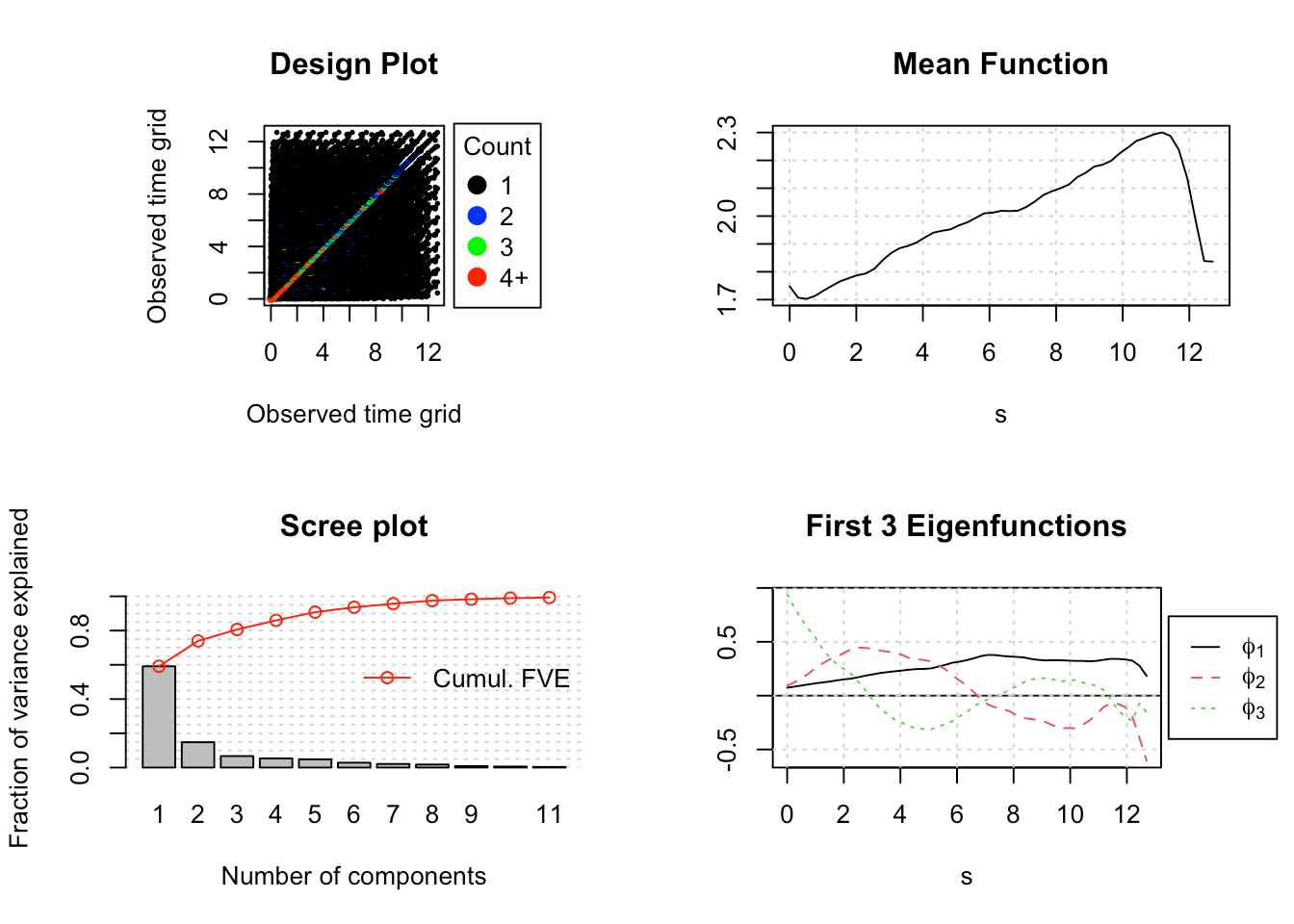We can obtain the exact estimates for the cumulative Fraction of Variance Explained by picking `cumFVE` out of the `FPCA` object.

```round(res_wages\$cumFVE,3)
##   0.591 0.739 0.806 0.860 0.908 0.936 0.957 0.975 0.983 0.989 0.993```

The following plot shows the smoothed curves estimated for the first two subjects. These are the same subject plotted in the third plot above. The circles indicate the input data. Everything looks pretty good here.

`CreatePathPlot(res_wages, subset=1:2, main = 'Estimated Paths for IDs 31 and 36'); grid()`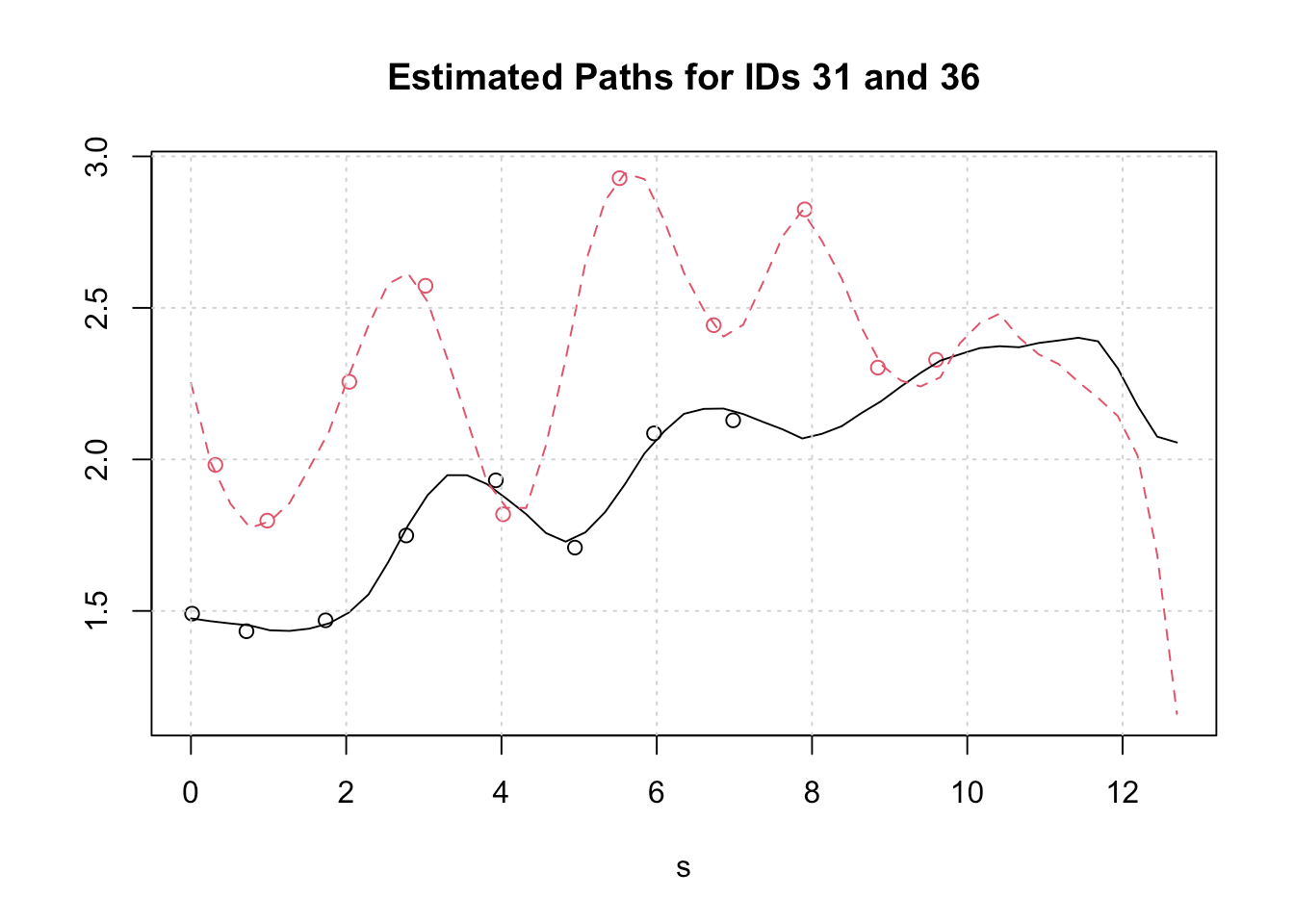But looking at just one more subject shows how quickly things can apparently go off the rails. The green curve for subject id 53 after 1.77 years is pure algorithmic imagination. Although there are several data points in the first two years, there is nothing thereafter.

`CreatePathPlot(res_wages, subset= 1:3, showMean=TRUE)`The value of the FPCA analysis lies in estimating the mean function and the covariance operator which are constructed from the pooled data, and not in predicting an individual paths.

The covariance surface is easily plotted with the help of the extractor function `GetCovSurface()` which fetches the time grid and associated covariance surface stored in the `FPCA` object. These are in the right format for a three dimensional, interactive `plotly` visualization. Rotating the plot and changing the viewing angle reveals quite a bit about the details of the estimated covariance surface.

```covS <- GetCovSurface(df3\$Wages,df3\$Exp)
x <- covS\$workGrid
Surf <- covS\$cov

fig <- plot_ly(x = x, y = x, z = ~Surf) %>%
z = list(show=TRUE,usecolormap=TRUE,
highlightcolor="#ff0000", project=list(z=TRUE))))

fig <- fig %>%
layout(scene = list(camera=list(eye = list(x=1.87, y=0.88, z=-0.64))))

fig```

The next plot, the Modes of Variation Plot shows the modes of variation about the mean for the first two eigenfunctions. The mean function is indicated by the red line. The dark gray shows the variation of the first eigenfunction, and the light gray shows the variation of the second. The plot indicates that all of the subjects begin their careers with similar entry level wages, start to diverge by their second year, reach peak variation around year eight and then begin to converge again by year eleven.

`CreateModeOfVarPlot(res_wages, main = "Modes of Variation of Eigenvectors")`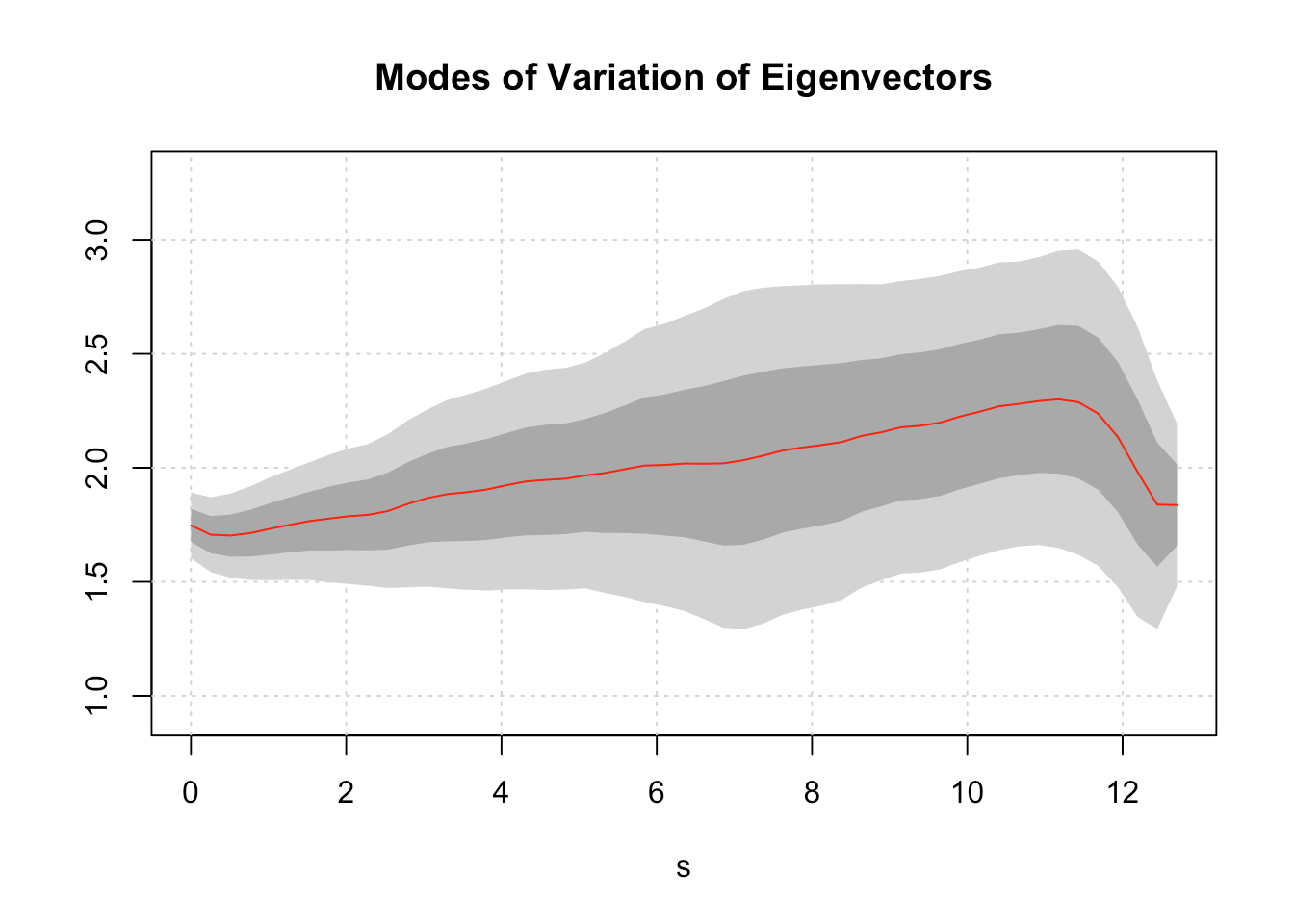### Some Conclusions

This short post neither presents a comprehensive view of functional principal components analysis, nor does it provide the last word on the `wages` data set. Nevertheless, juxtaposing the highly visual but traditional exploratory analysis conducted by the authors of the `brolgar` package with a basic FPCA look does provide some insight into the promise and pitfalls of using FPCA to explore sparse, longitudinal data sets.

On the promise side:

1. FDA offers a global perspective that facilitates thinking about an individual subject’s time path as a whole. For the `wages` data set, we see individual trajectories developing in a wages/time space that can be parsimoniously represented, analyzed and compared.

2. FPCA works with very sparse data, does not require the same number of observations for each subject, and does not demand that the observations be taken at the same time points.

3. There is really no concept of missing data per se, and no need for data imputation. The amount of information required to represent a subject can vary over a wide range.

As to the pitfalls:

1. As with plain old multivariate PCA, eigenvectors may not have any obvious meaning, and trajectories in an abstract space may be difficult to interpret.

2. There is really no way to avoid the missing data daemon. The `wages` data set shows the sensitivity of FPCA trajectories to both the number and the locations of the observed data points. It is not possible to reconstruct individual trajectories for which there are too few observations.

That’s all for now. Thank you for following along.